# Learning to Predict Vehicle Trajectories with Model-based Planning CoRL 2021

Hong Kong University of Science and Technology

### Abstract

Predicting the future trajectories of on-road vehicles is critical for autonomous driving. In this paper, we introduce a novel prediction framework called PRIME, which stands for Prediction with Model-based Planning. Unlike recent prediction works that utilize neural networks to model scene context and produce unconstrained trajectories, PRIME is designed to generate accurate and feasibility-guaranteed future trajectory predictions, which guarantees the trajectory feasibility by exploiting a model-based generator to produce future trajectories under explicit constraints and enables accurate multimodal prediction by using a learning-based evaluator to select future trajectories. We conduct experiments on the large-scale Argoverse Motion Forecasting Benchmark. Our PRIME outperforms state-of-the-art methods in prediction accuracy, feasibility, and robustness under imperfect tracking.

(PRIME had been ranked 1st on the Argoverse Motion Forecasting Challenge until March 2021.)

### Motivation & Key idea

In traffic scenarios, most vehicles operate under their inherent kinematic constraints (e.g., non-holonomic motion constraints for vehicles) while in compliance with the road structure (e.g., lane connectivity, static obstacles) and semantic information (e.g., traffic lights, speed limits). All these kinematic and environmental constraints explicitly regularize the trajectory space.
However, most existing future prediction approaches model traffic agents as points and produce sequences of future positions without constraints. Such constraint-free predictions may be incompliant with kinematic or environmental characteristics, which gives rise to massive uncertainty in the predicted future states. Consequently, the downstream planning module would inevitably undergo some extra burdens and even the "freezing robot problem."
Moreover, the recent learning-based prediction models follow the typical paradigm of generating trajectory predictions by network regression that highly relies on long-term tracking results. But for some dense driving scenarios where the target would be momently occluded or suddenly appears within the sensing range, tracking results are discontinuous or not accumulated enough. The prediction accuracy would degrade under such imperfect tracking cases.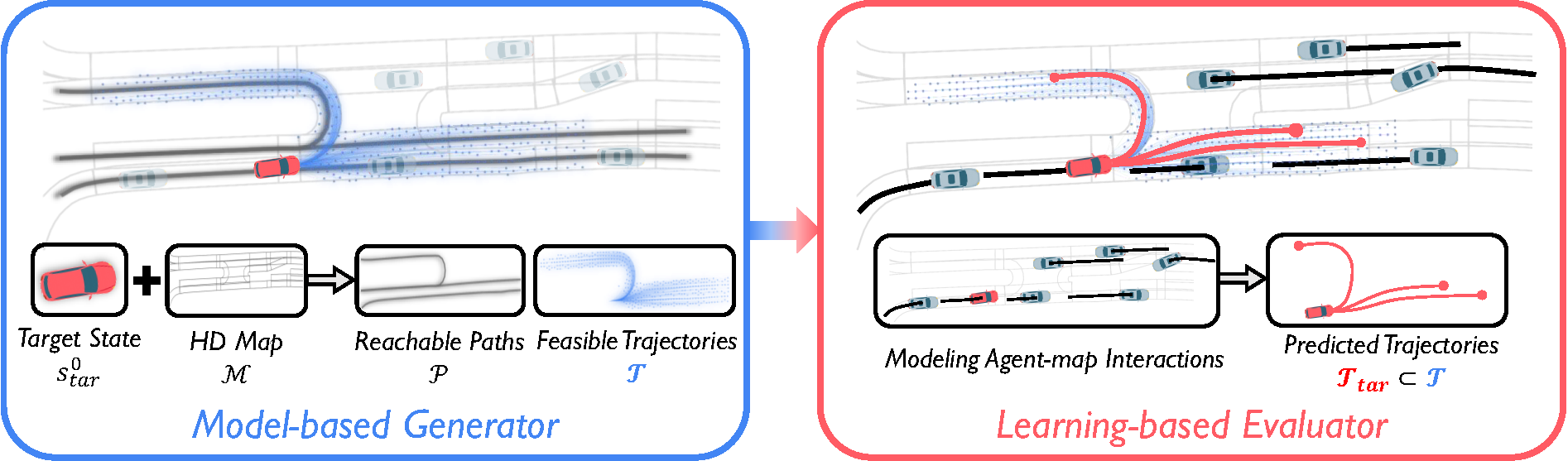Toward overcoming these challenges, we propose a novel prediction architecture called PRIME. The critical idea is to exploit a model-based motion planner as the prediction generator to sample feasible future trajectories under explicit constraints, together with a deep neural network as the prediction evaluator to model implicit interactions and select future trajectories by scoring. The novel architecture contributes to accurate, feasible, and robust trajectory predictions.
More specifically, the model-based generator (left) which samples the target's feasible future trajectories $$\mathcal{T}$$ by taking its real-time state $$\mathbf{s}_{tar}^0$$ and the map $$\mathcal{M}$$, while explicitly imposing kinematical and environmental constraints to guarantee trajectory feasibility; the learning-based evaluator (right) which receives the feasible trajectories $$\mathcal{T}$$ and all observed tracks $$\mathcal{S}$$ to model the implicit interactions among all traffic agents, and selects a final set of feasible trajectories $$\mathcal{T}_{tar}\subset\mathcal{T}$$ as the prediction result.

### Framework Overview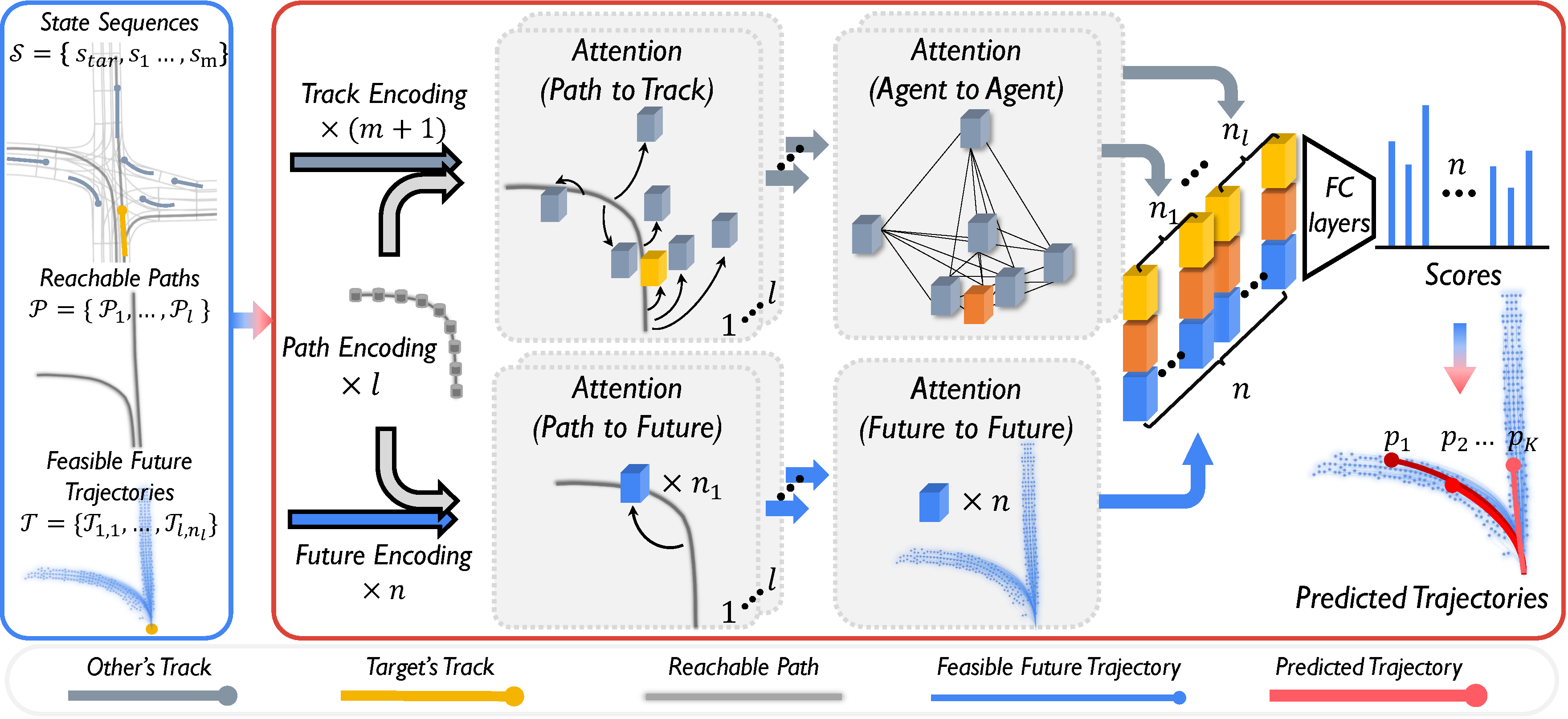The model-based generator searches reachable paths $$\mathcal{P}$$ through the map with Depth-First-Search and samples a set of feasible future trajectories $$\mathcal{T}$$ with the Frenet Planer. This part is detailed in our paper.
The learning-based evaluator first encodes scene context given by $$(\mathcal{P}, \mathcal{T}, \mathcal{S})$$, including $$l$$ paths in $$\mathcal{P}$$, $$(m+1)$$ history tracks in $$\mathcal{S}$$ and $$n$$ future trajectories in $$\mathcal{T}$$. The implicit agent-map interactions are learned in the subsequent attention modules: P2T and P2F propagate the spatial information of each reference path $$\mathcal{P}_i$$ into history tracks and corresponding future trajectories, and A2A takes track tensors from P2T to capture the multi-agent interactions. As the path-based Frenet coordinate is used in our dual spatial representation, P2T, P2F, and A2A operate for each path, while F2F fuses all the future trajectories processed by P2F to obtain a global understanding for the reachable space. Subsequently, each feasible trajectory $$\mathcal{T}_{j,k}$$ could query its track tensor $$\mathbf{X}_j(\mathbf{s}_{tar})$$ from P2T, interaction tensor $$\mathbf{Y}_j(\mathbf{s}_{tar})$$ from A2A and future tensor $$\mathbf{Z}(\mathcal{T}_{j,k})$$ from F2F, and it is scored by feeding the concatenation of these tensors to fully-connected layers. Finally, the evaluator ranks all feasible future trajectories in $$\mathcal{T}$$ by scoring and outputs a final set of $$K$$ predicted trajectories.

### Quantitative Results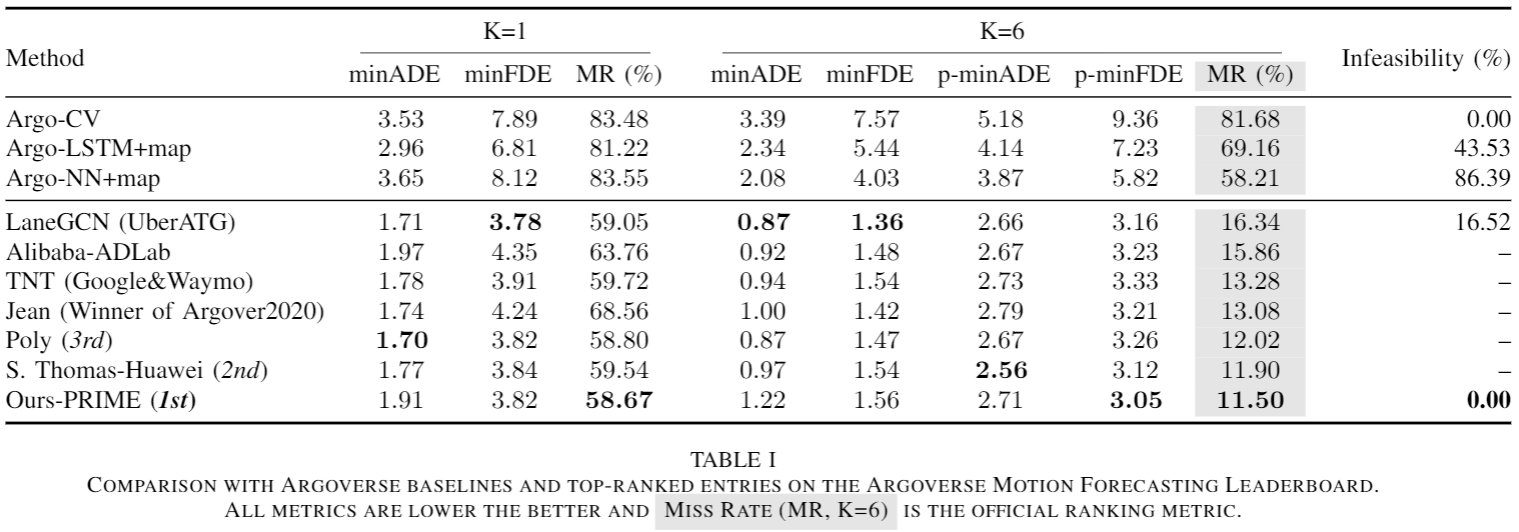### Qualitative Results

Qualitative results under various scenarios on the Argoverse validation set. The model-based generator produces the set of future trajectories $$\mathcal{T}$$ (blue) with feasibility guaranteed, which well regularize the target vehicle's future trajectory space. The learning-based evaluator selects $$K$$ trajectories from $$\mathcal{T}$$ as multimodal prediction results (red), and the depth of red indicates their probability.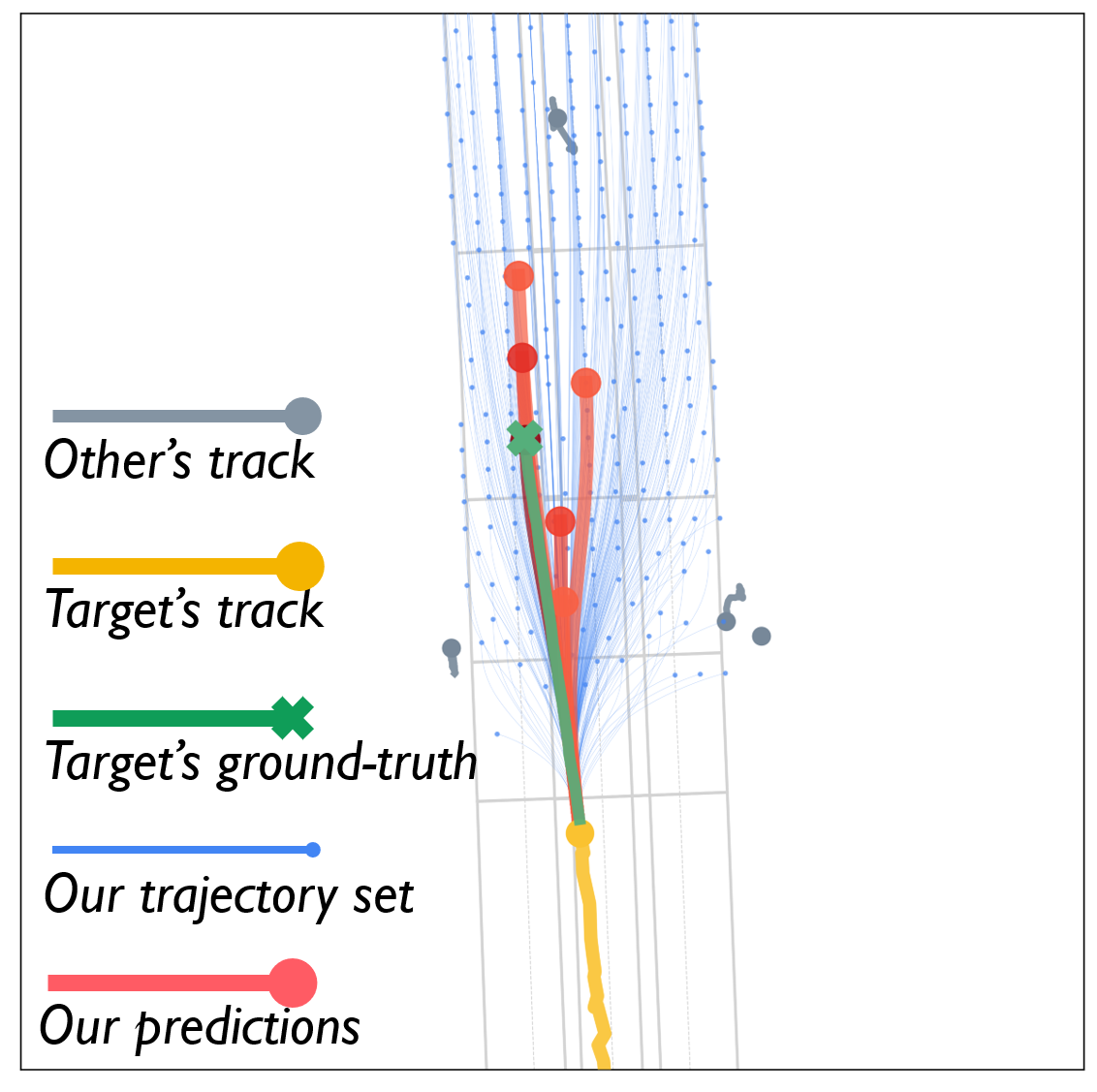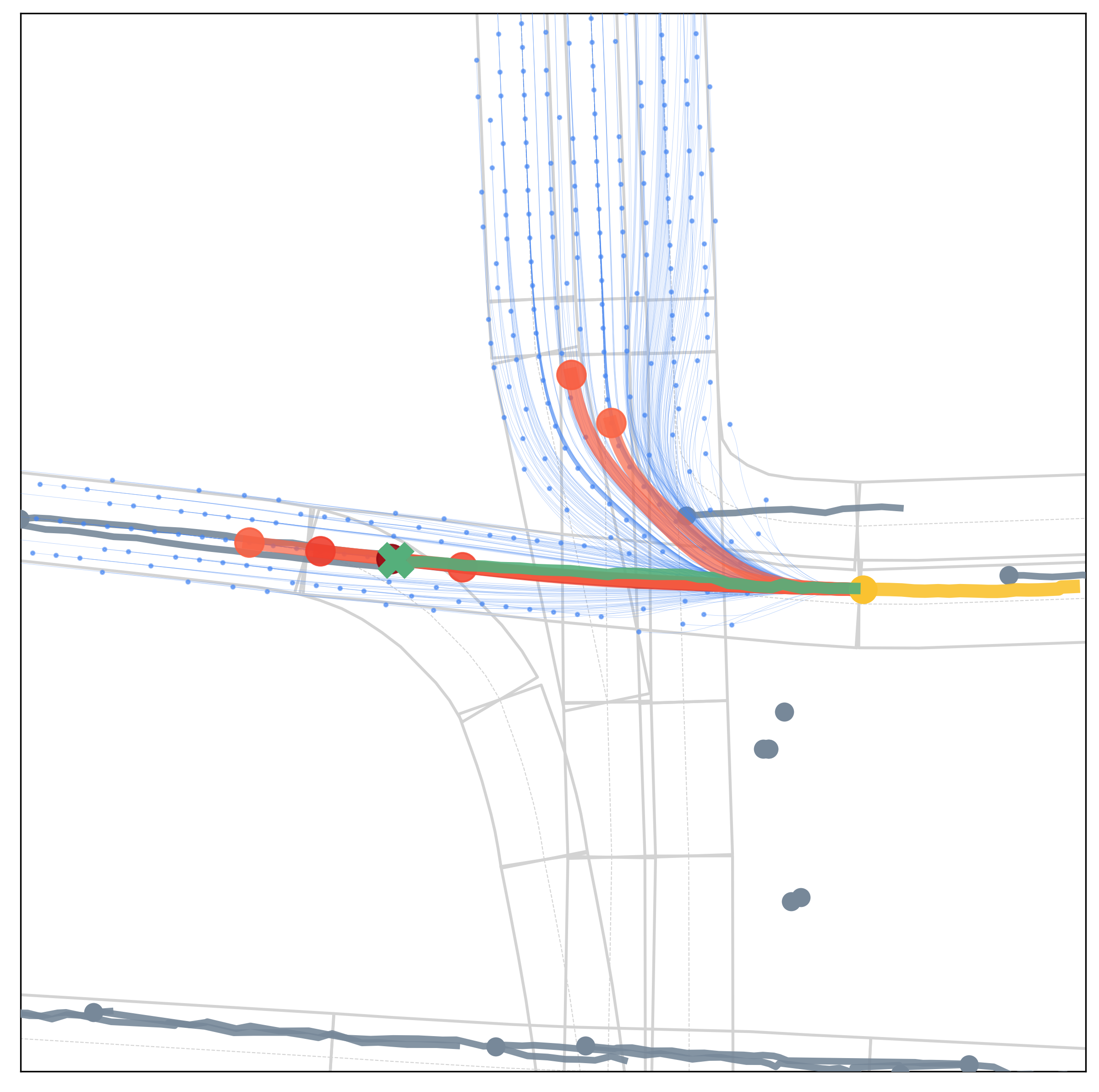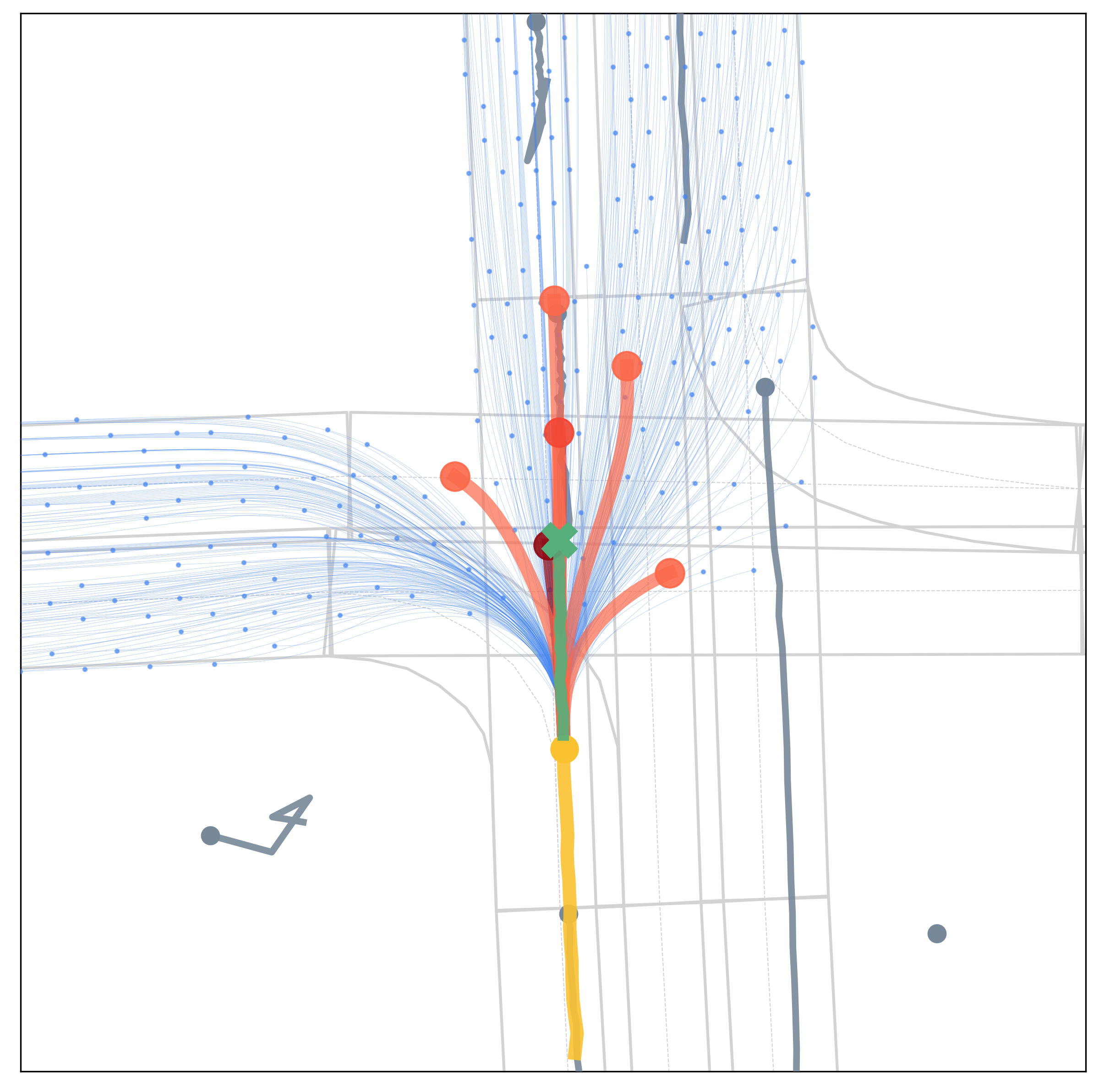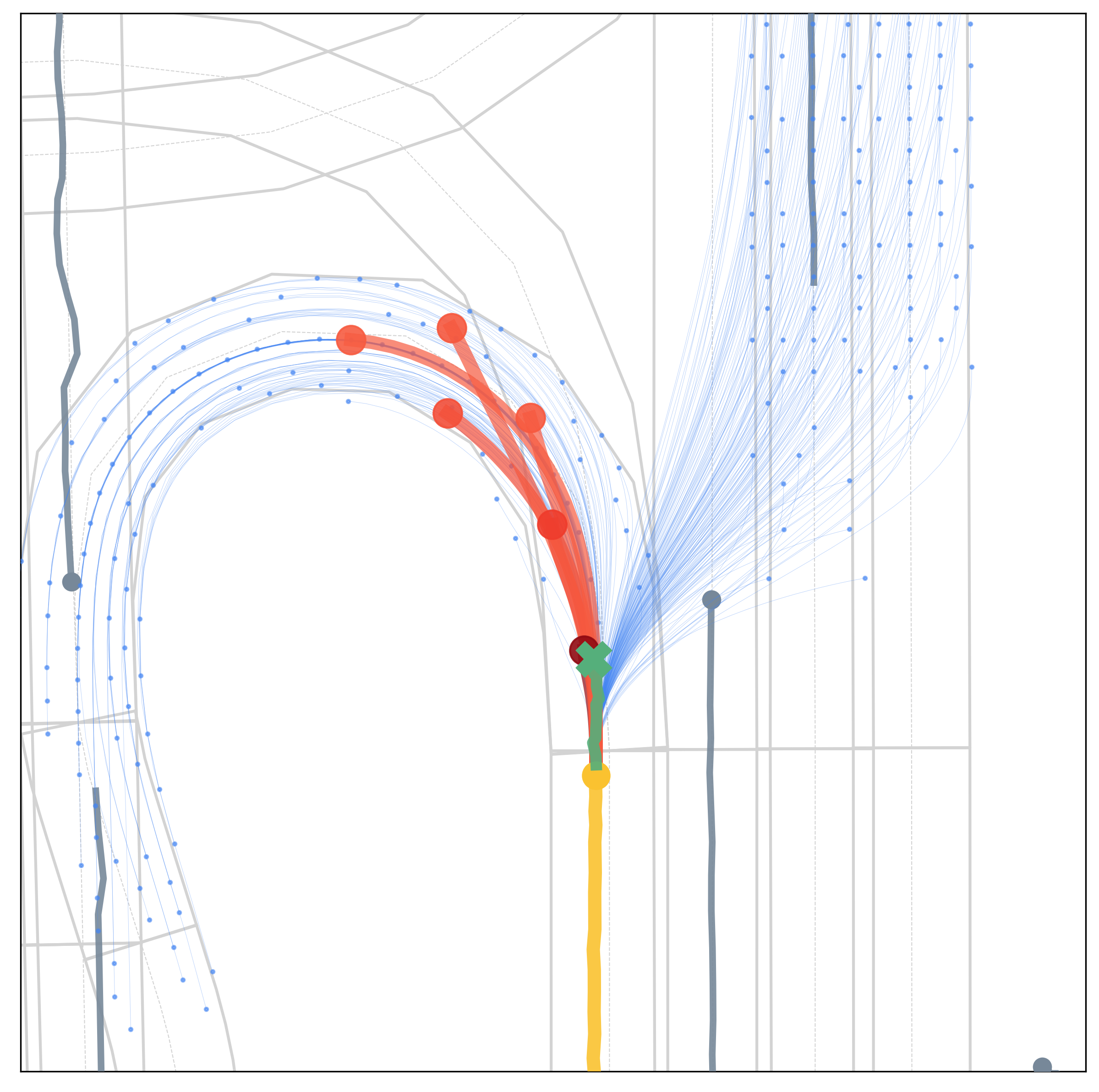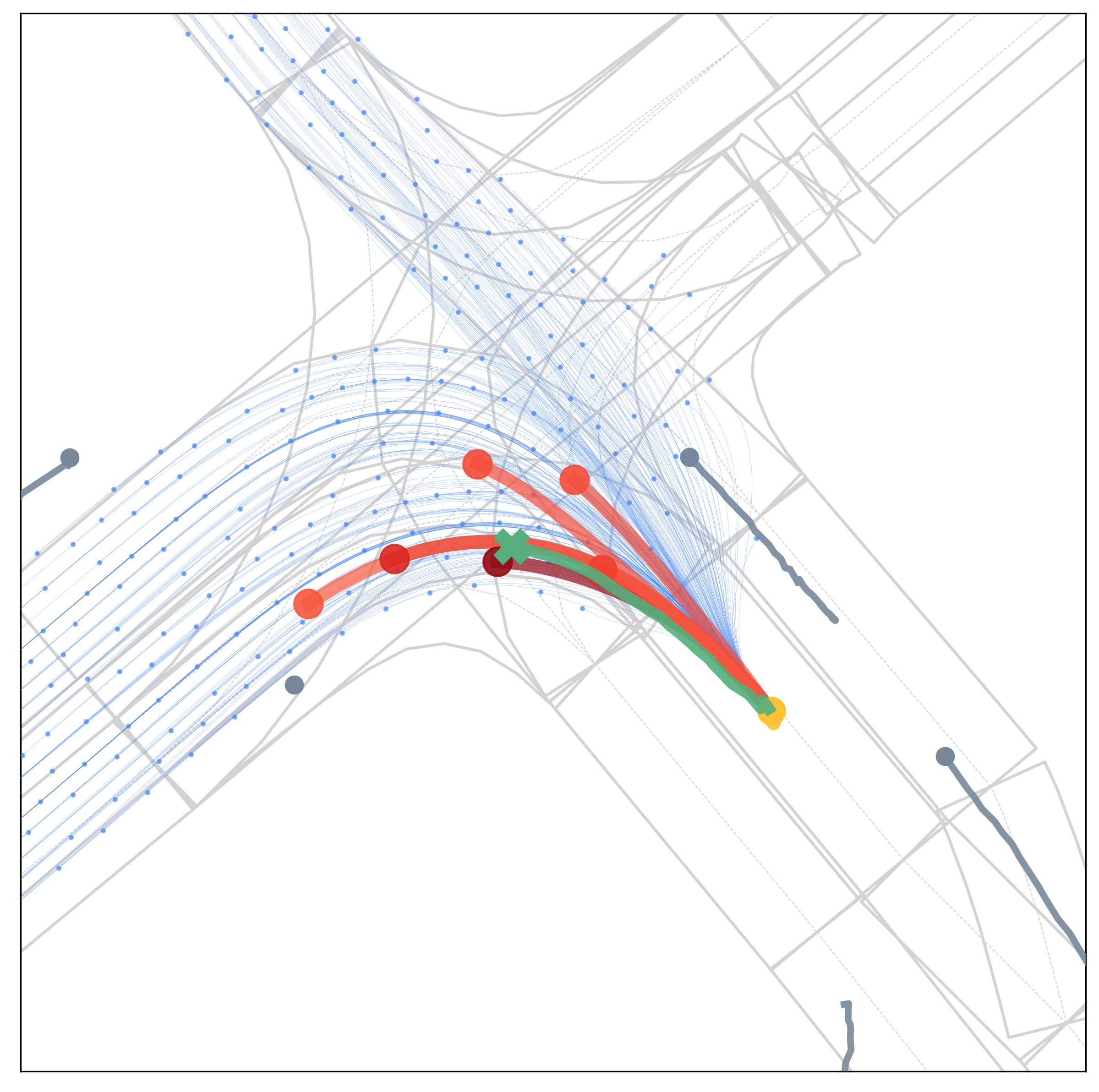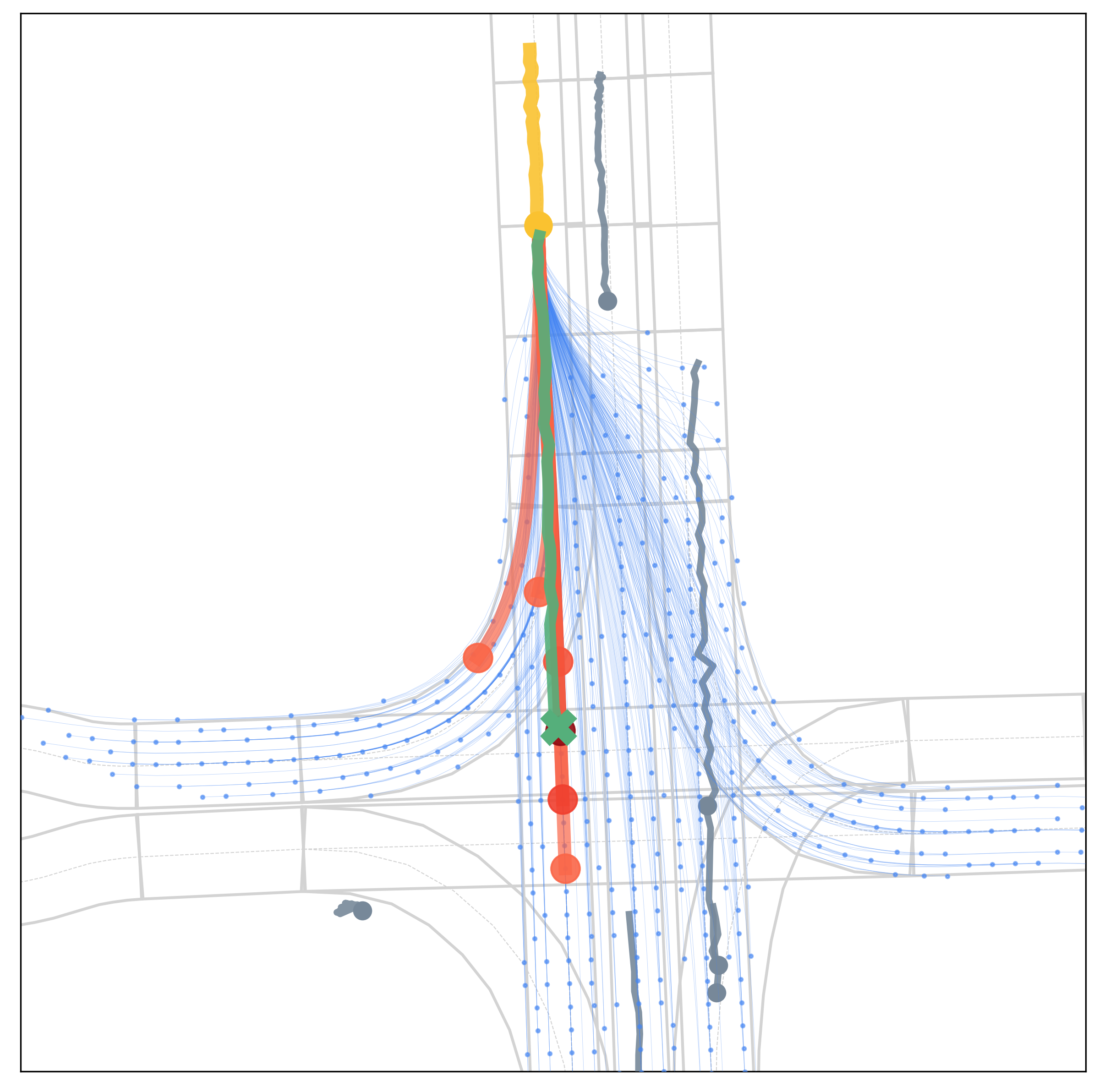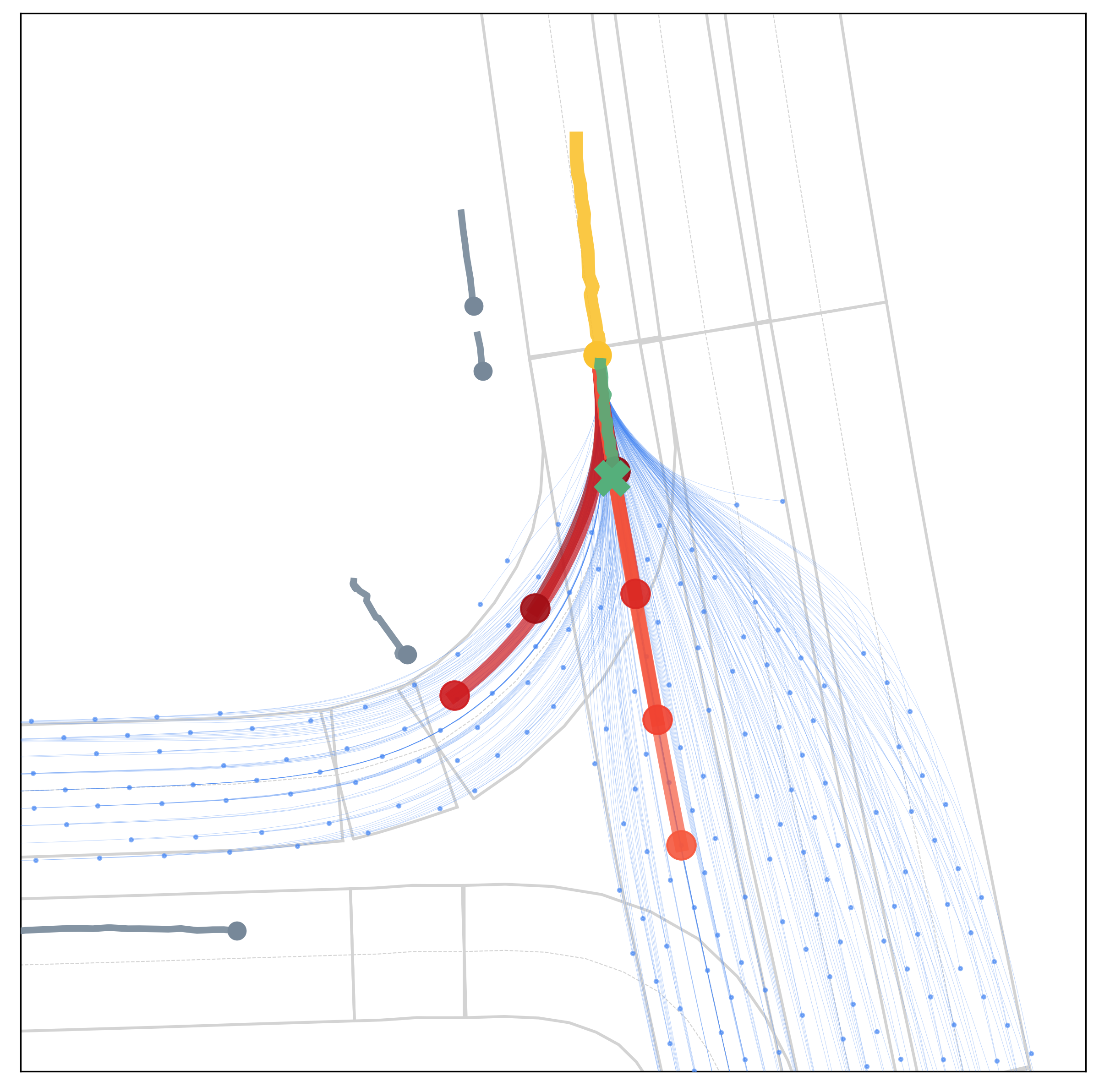### [Supp. 1] Comparison with Fully Learning-based Prediction

Compared with the mainstream learning-based methods that generate unconstrained trajectory predictions by neural networks, the main difference of our proposed PRIME framework is to explicitly constrain the prediction space and thereby ensure trajectory feasibility. Here, we use LaneGCN as a representative for the typical fully learning-based prediction models, and among the current state-of-the-art methods, it is open-source. We demonstrate some common failures of kinematically and environmentally infeasible predictions in the following.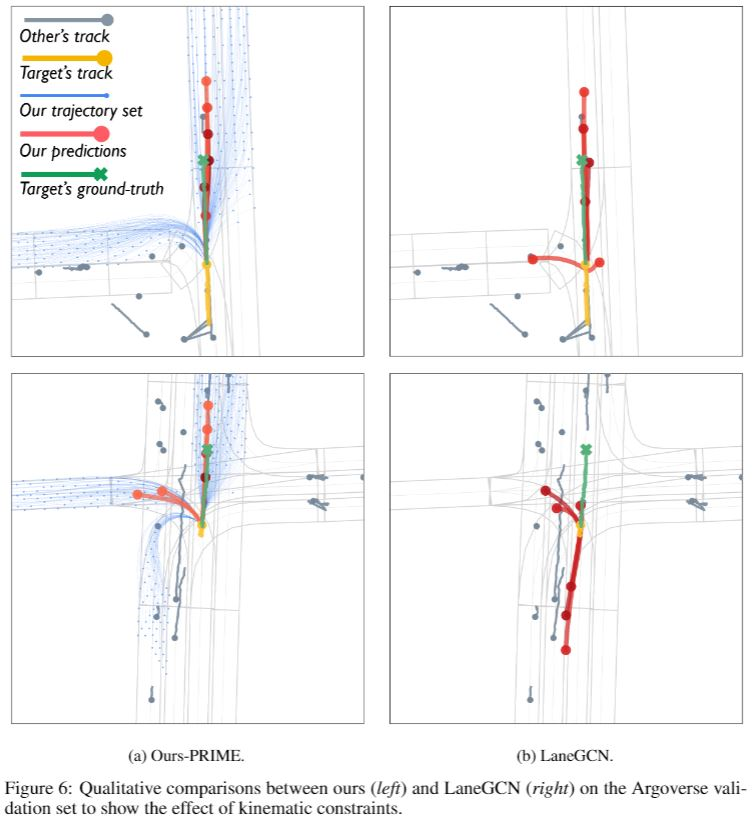Due to kinematic constraints, vehicles cannot take a sudden turn at high speed (1st-row in Fig. 6), or reverse the moving direction (2nd-row in Fig. 6). Also, the prediction results of turning with across lane boundaries (1st-row in Fig.7), or heading towards reverse lanes (2nd-row in Fig.7) are incompliant with environmental constraints. Moreover, the counter-intuitive bidirectional trajectories predicted by LaneGCN (2nd-row in Fig.7) also reveal that the fully learning-based prediction relies on relative long-range tracks for regressing trajectories, but it may degrade under short-range tracks.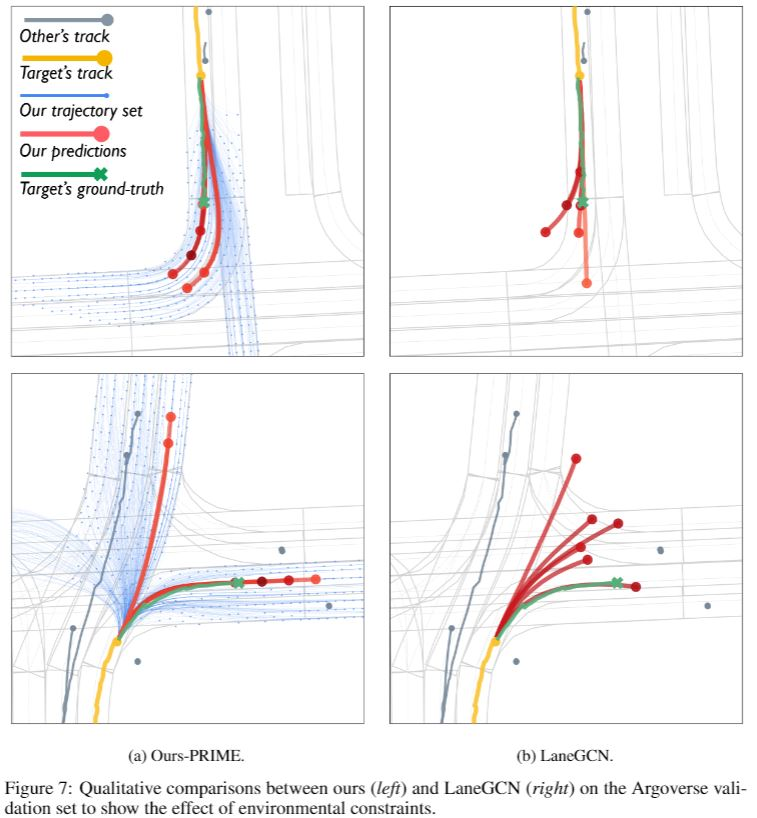In some of the above examples, although it looks PRIME and LaneGCN show comparable performance when evaluated by minADE$$_6$$ and minFDE$$_6$$, their impacts on the downstream planning differ a lot. The infeasible trajectories generated by LaneGCN bring massive uncertainty in the predicted future states, which would cause redundant burdens for an autonomous vehicle to make decisions and motion plans. Especially in dense traffic where multiple surrounding vehicles need to be predicted, the negative impact of infeasible predictions would be further aggravated. By contrast, PRIME regularizes the future trajectory space (blue) by given constraints and thus makes accurate and reasonable future predictions (red).

### [Supp. 2] Impacts Caused by Defect Data

Although Argoverse is one of the most recognized benchmarks for trajectory prediction due to its high-quality trajectory and map annotation, some of its ground truth trajectories are not completely correct. The common issues result from the tracking method used for annotating the data, including position oscillation (Fig. 8(a)) and id switch (Fig. 8(b)) that the ground truth trajectory is suddenly switched to a neighboring agent. Such defect cases would lead to worse performance indicators (ADE/FDE-based metrics) of our method in the quantitative evaluation, but it is evident that the smooth trajectories predicted by PRIME are more realistic and reasonable.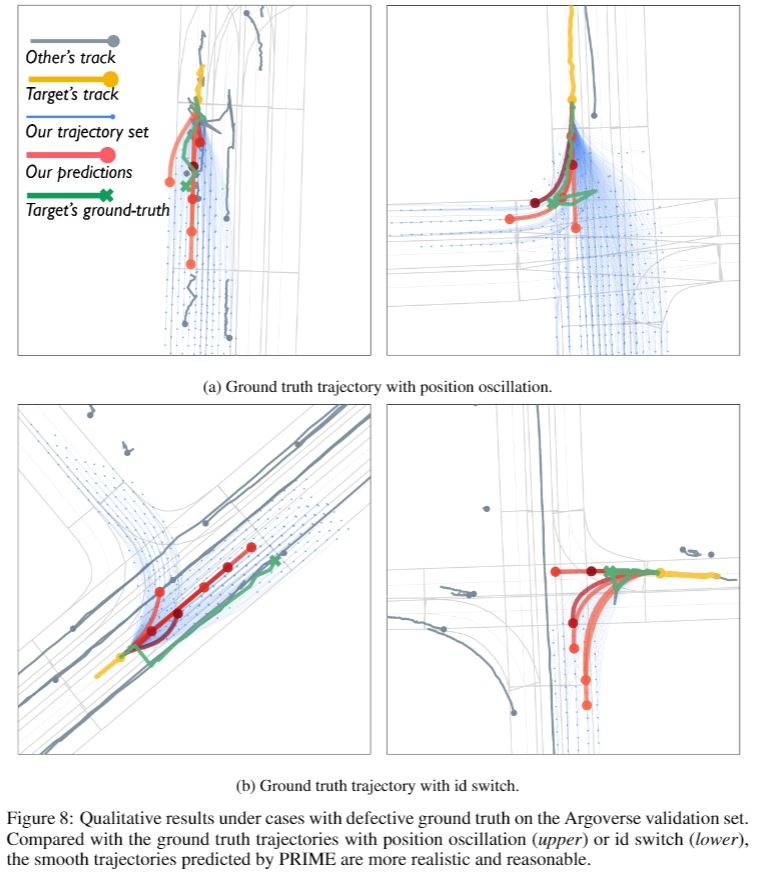### [Supp. 3] Failure Cases

We demonstrate the failure cases of our method on the Argoverse validation set in Fig. 9. The failures are mostly related to the estimation deviation for the target vehicle's current state $$\mathbf{s}_{tar}^0$$. Although the sampling-based strategy in our generator could compensate for inaccurate state estimation to some extent, estimating the heading and velocity from sequences of centroid positions would be intractable when there exists serious data noise. For example, the position oscillation of a short-distance history track would make the heading direction hard to estimate, as shown in Fig. 9(a). As a result, the ground truth trajectory locates out of the resulted prediction space's span range. When the position sequence vibrates too much, the accuracy of velocity estimation would even be affected. As exemplified in Fig. 9(b), the future trajectory space does not cover the ground truth trajectory due to the inaccurate estimation for the target's low velocity, leading to a relatively large displacement error in the prediction results.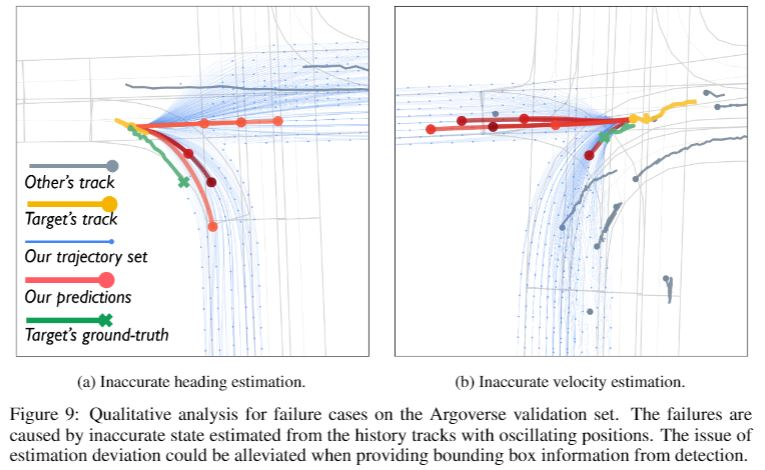Nonetheless, the accuracy of state estimation could be improved by incorporating more information. For instance, the vehicle's bounding box given by detection provides geometry information in addition to discrete positions, which would enable more robust and accurate state estimation for prediction targets.

### Runtime Analysis

The inference frequency of our prediction framework depends on the scene complexity, sampling density, and computing power. Running with Intel i7-7820X, the generation of a single trajectory with a single thread spends 0.1~0.2 ms on average. With each trajectory sample produced independently, the model-based trajectory generator could be highly parallelized to provide full coverage to the future prediction space with satisfactory real-time performance. For the learning-based evaluator, it is implemented by a lightweight network with only 1.02 million parameters. Its inference time on NVIDIA 2080TI is 8~12 ms. Overall, the whole framework of PRIME could well satisfy the real-time requirements for autonomous driving.

(Note: The current C++ implementation of the model-based part has a 1/10 runtime of the previous version implemented by Python. i.e., sampling 1k trajectories on single thread costs ~10ms.)

### BibTeX

@inproceedings{song2021learning,
title={Learning to Predict Vehicle Trajectories with Model-based Planning},
author={Haoran Song and Di Luan and Wenchao Ding and Michael Y Wang and Qifeng Chen},
booktitle={5th Annual Conference on Robot Learning },
year={2021},
}